# Recycled Meter Artwork

Battery powered artwork made from recycled ammeter to hang on your wall

BeginnerShowcase (no instructions)2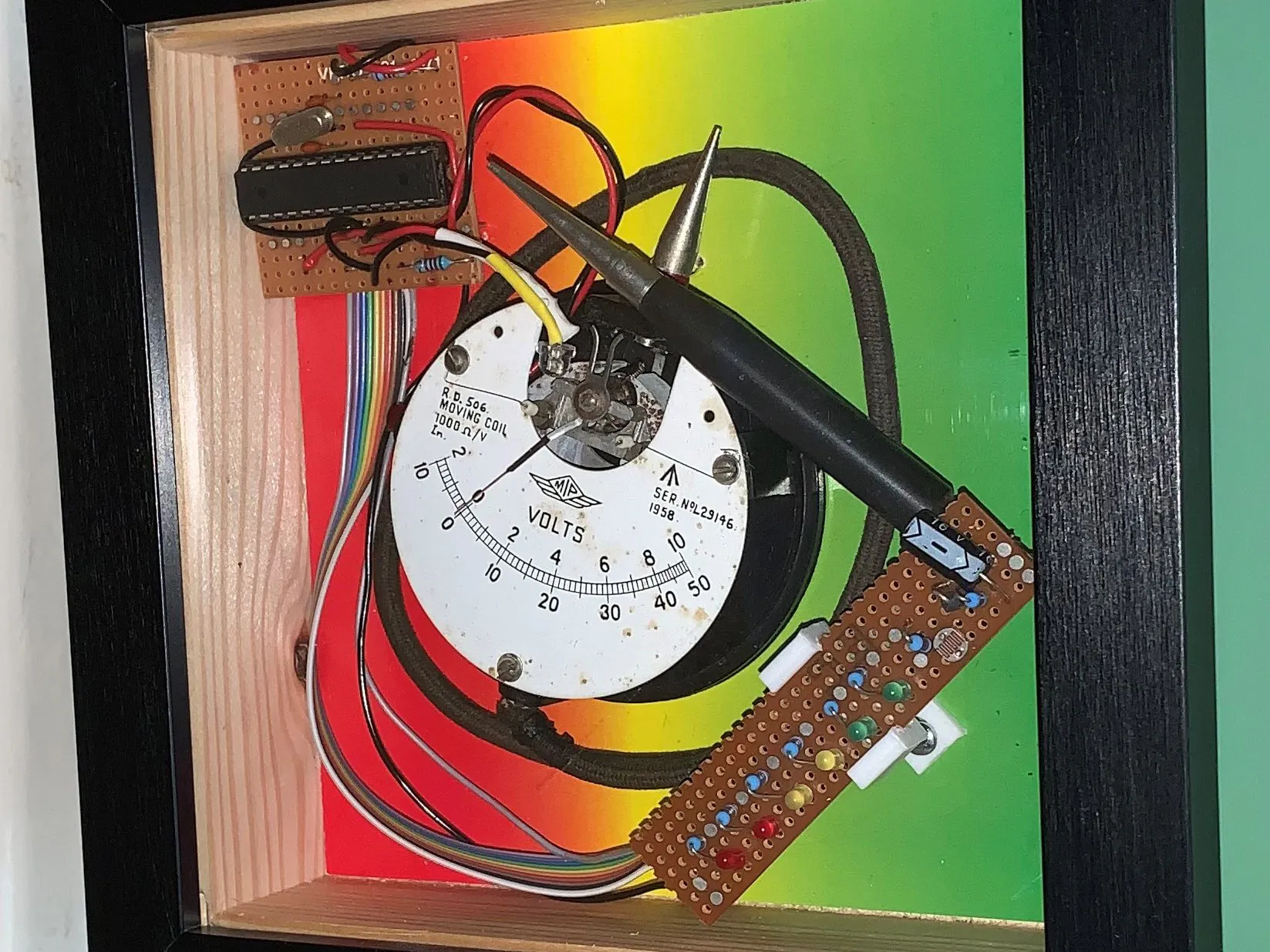## Things used in this project

### Hardware componentsArduino UNO & Genuino UNO Replaced with small circuit board to which the Atmel 328 is transferred
×1×1Jumper wires (generic)
×15 mm LED: Red
×2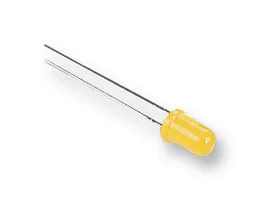5 mm LED: Yellow
×2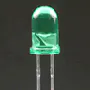5 mm LED: Green
×2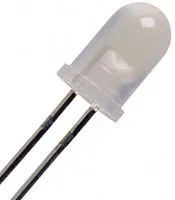High Brightness LED, White
×1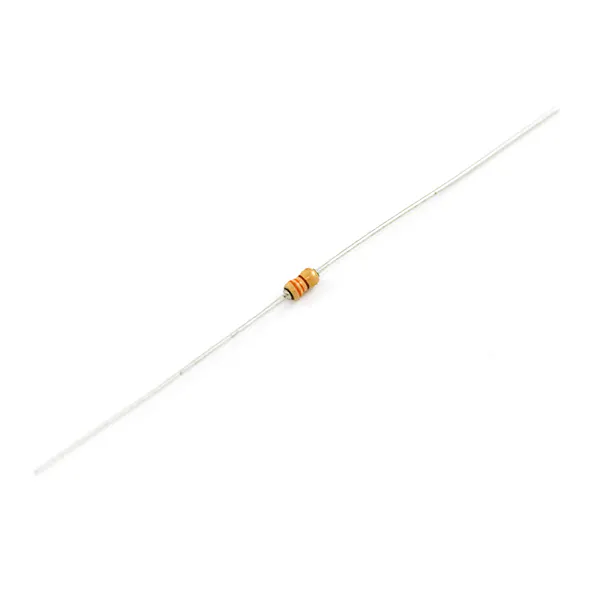Resistor 330 ohm
×7Resistor 10k ohm
×2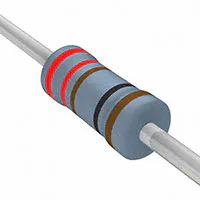Resistor 221 ohm
×1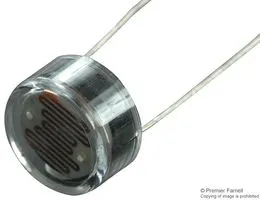LDR, 1 Mohm
×1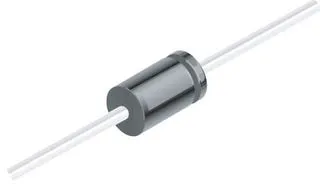1N4007 – High Voltage, High Current Rated Diode
×1Capacitor 220 µF
×1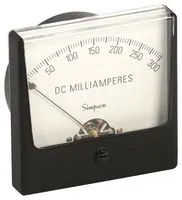Analog Panel Meter, Black Spade Type Pointer This was actually a recycled meter which was modified to expose the mechanism
×1

## Schematics

Runs the basic animation of the meter and the light display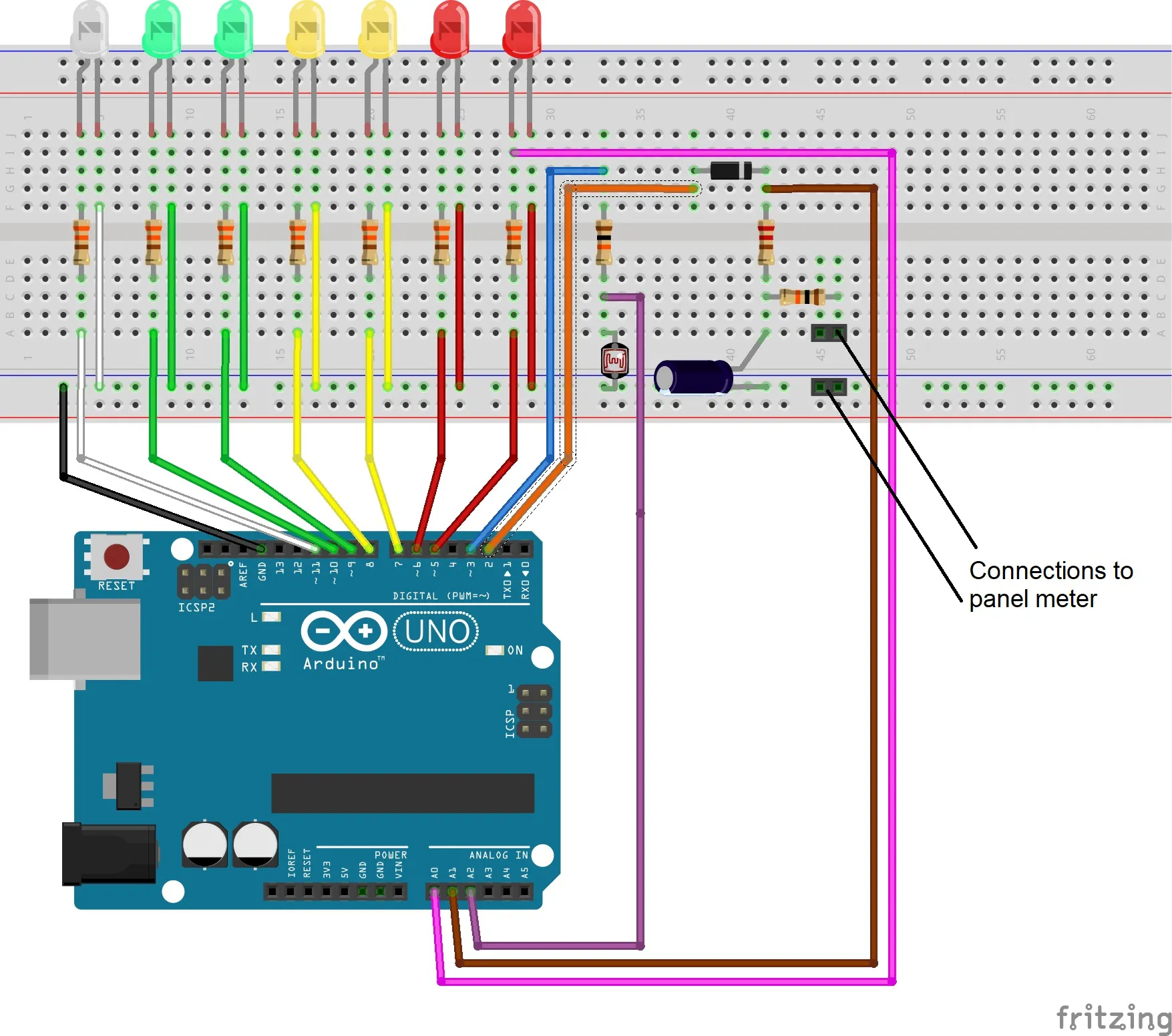### Schematic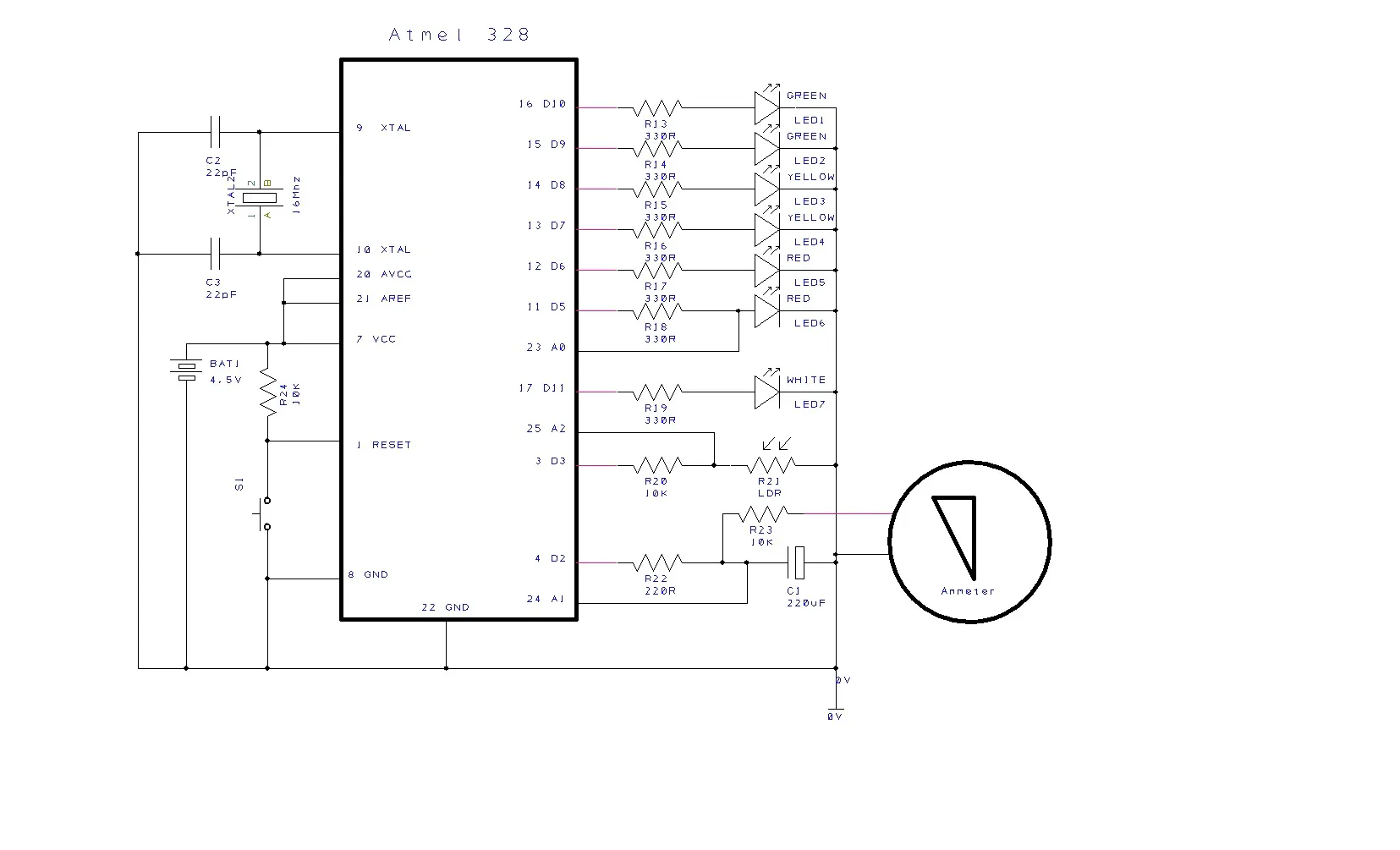## Code

### Wall_Meter_Display.ino

Arduino
Runs a basic light display and is intended to run with very low power consumption for battery operation
```#include <Narcoleptic.h>

/*
WallDisplay - Traffic light
Creates a basic traffic light display for a wallmount
*/
int voltref = 0;    // hold reference voltage level for low voltage calculations
int demoCount = 0;   // used to run the demo section when first powered up
int Direction = 1;   // sets direction either 0 or 1
int SetDelay = 1; //0=standard delay, 1=NarcoDelay, low power and no serial port usage
unsigned long randInit = 20;  // limits of random number generator, set low for testing
int LDR;          // voltage measured on LDR
int darkLimit = 1000;  // ldr reading for its dark
int lightLimit = 750;  // ldr reading for its light
int timeOfDay = 1;  // time of day set to gloaming so that power on and reset runs full display  0 = night, 1 = gloaming, 2=day
int lightChange = 0;  // count of contiguous light LDR samples
int gloamingChange = 0;   // count of contiguous gloaming LDR samples
int darkChange = 0;   // count of contiguous dark LDR samples
int randLimit = 20;  // limits of random number generator, set low for testing
int delayPeriod = 4000; //inital delay period just in case its needed
int summer = 14970;  // length of longest day in samples
int winter = 7035;  // length of shortest day in samples
int longDayCount = 0;  // approximate length of day in displays
int shortDayCount = 0;  // approximate length of day in displays
int maxDay = 0;  // previous length of light in display cycles
int delayDayCount = 21; // number of display cycles to defer in the morning based on previous days estimated length
int capacitor=2 // pin applying power to capacitor
int LDRpower=3 // pin applying power to ldr
int red1=5;   // 1st red led
int red2=6;   // 2nd red led
int amber1=7;   // 1st amber led
int amber2=8;   // 2nd amber led
int green1=9;   // 1st green led
int green2=10;   // 2nd green led
int whiteled=11; // white led to illuminate meter

// ---------------------------------------------------------------------------------------
// the setup function runs once when reset it pressed or power the board
void setup() {
// initialize digital output pins
//   pin A1
//   pin A2
//   pin A3
pinMode(capacitor, OUTPUT);
pinMode(LDRpower, OUTPUT);
pinMode(red1, OUTPUT);
pinMode(red2, OUTPUT);
pinMode(amber1, OUTPUT);
pinMode(amber2, OUTPUT);
pinMode(green1, OUTPUT);
pinMode(green2, OUTPUT);
pinMode(whiteled, OUTPUT);

longDayCount = (summer - winter) / randLimit;
shortDayCount = winter / randLimit;

randInit = LDRvoltage();
randomSeed(randInit);          // randomise on light level
if (SetDelay == 0)     // only run serial output if standard not narco delay
Serial.begin(9600);
}

// ---------------------------------------------------------------------------------------
// the loop function runs over and over again forever
void loop() {
int newTimeOfDay;
// only run routine if random comes up otherwise sleep

randInit = random(randLimit);
SerialSub(SetDelay, "Random ", randInit);
// ---------------------------------------------------------------------------------------

if (delayDayCount < 20)   //  count down non running of display after dawn when no one will be watching
{
delayDayCount++;
randInit=0;    // extend non running after dawn
SerialSub(SetDelay, "Delay ", demoCount);
}
// ---------------------------------------------------------------------------------------
if (demoCount < 10)   //  demo and test routine runs every time after power on or reset
{
demoCount++;
randInit=1;    // fudge for test functions
SerialSub(SetDelay, "Demo ", demoCount);
}
// ---------------------------------------------------------------------------------------
if (randInit == 1)
{
voltref = refVoltage();    // get the reference voltage
LDR = LDRvoltage();   // get light level
newTimeOfDay = dayOrNight();
if (timeOfDay != newTimeOfDay)
timeOfDay = newTimeOfDay;   // swap to new time of day if theres an update
SerialSub(SetDelay, "timeOfDay", timeOfDay);
if (timeOfDay == 0)    // if night then just wait for 4 or 8 seconds
{
if (SetDelay == 0)
delayPeriod = 4000;   // if standard sleep then fixed to 4 seconds
else
delayPeriod = 8000;  // if narco then sleep extra time
}
else
{
Subloop();    // This breakout runs the display
delayPeriod = 4000;
}
}
Ndelay(SetDelay, delayPeriod); //  Delay for 'delayPeriod' seconds approximately
}

// ---------------------------------------------------------------------------------------
int LDRvoltage() {
digitalWrite(LDRpower, HIGH);     // activate LDR to check light levels
digitalWrite(LDRpower, LOW);
}

// ---------------------------------------------------------------------------------------
// Estimate whether it is day, gloaming or night. The unit has to be in one state for at least four iterations before a state change is registered
// the sequence is light, gloaming, dark, light.
int dayOrNight() {
int dayLight;
dayLight = timeOfDay;
if (lightChange > maxDay)
maxDay = lightChange;
if (LDR > darkLimit)   // calulate continuous state
{
lightChange = 0;
gloamingChange = 0;
darkChange++;
}
else if (LDR < lightLimit)
{
lightChange++;
gloamingChange = 0;
darkChange = 0;
}
else
{
lightChange = 0;
gloamingChange++;
darkChange = 0;
}
if (lightChange >10)   // after state the same for four iterations return a change
dayLight = 2;
else if (gloamingChange > 10)
dayLight = 1;
else if (darkChange > 10)
dayLight = 0;
if (timeOfDay == 0 and dayLight==1) // do not allow change from dark to gloaming
dayLight = 0;
SerialSub(SetDelay, "DayOrNight", dayLight);
return dayLight;
}

// ---------------------------------------------------------------------------------------
// get reference voltage for low battery compensation
int refVoltage() {
digitalWrite(red1, HIGH);
digitalWrite(red2, LOW);
}

// ---------------------------------------------------------------------------------------
// Return capacitor voltage
int capVoltage() {
}

// ---------------------------------------------------------------------------------------
// routine to charge capacitor
void chargeCap() {
if (timeOfDay == 1)
digitalWrite(whiteled, HIGH);     // activate meter illumination
Ndelay(SetDelay, 300); // wait for 300 milliseconds to attract attention
do {
digitalWrite(capacitor, HIGH);  // start to charge capacitor
Ndelay(SetDelay, 100); // wait for 100 milliseconds for capacitor to charge
} while (capVoltage()<800);
Ndelay(SetDelay, 200); // wait for 200 milliseconds for capacitor to display
digitalWrite(capacitor, LOW);  // stop charging
}

// ---------------------------------------------------------------------------------------
// routine to reverse directiion setting
void reverseDirection() {
if (Direction == 1)    // reverse previous light direction
{
Direction = 2;
}
else
{
Direction = 1;
}
}
// ---------------------------------------------------------------------------------------
// Main display loop
void Subloop() {

// Start by charging capacitor
chargeCap();

// Reverse previous direction to keep variations
reverseDirection();

// run light display until capacitor runs down
// routine only runs LEDs if gloaming when viewers are likely to see it
do {
if (Direction == 1 and timeOfDay == 1)
{
Traffic1();    // run forward light run
}
if (Direction == 2 and timeOfDay == 1)
{
Traffic2();    // run reverse light run
}
if (timeOfDay == 2)
Ndelay(SetDelay, 1000); //add time delay omitted due to not running lights
if (random(20) == 1) // Add restart to cap voltage to give random extended displays
{
chargeCap();
reverseDirection();
}
}  while (capVoltage() > 50);

digitalWritewhiteled, LOW);      // turn off meter illumination when done
}

// ---------------------------------------------------------------------------------------
// One of two LED driver routines which switch on LEDs according to the voltage on the capacitor
void Traffic1()
{
int light = capVoltage();

if (light > 60)
{
digitalWrite(red1, HIGH);   // Turn on red
Ndelay(SetDelay, 100); // wait for 100 milliseconds
}
if (light > 80)
{
digitalWrite(red2, HIGH);   // turn on red
Ndelay(SetDelay, 100); // wait for 100 milliseconds
}
if (light > 120)
{
digitalWrite(amber1, HIGH); // Turn on anber
Ndelay(SetDelay, 100); // wait for 100 milliseconds
}
if (light > 200)
{
digitalWrite(amber2, HIGH);   // Turn on amber
Ndelay(SetDelay, 100); // wait for 100 milliseconds
}
if (light > 320)
{
digitalWrite(green1, HIGH);   // turn on green
Ndelay(SetDelay, 100); // wait for 100 milliseconds
}
if (light > 580)
{
digitalWrite(green2, HIGH); // Turn on green
Ndelay(SetDelay, 100); // wait for 100 milliseconds
}
digitalWrite(red1, LOW);
Ndelay(SetDelay, 100);
digitalWrite(red2, LOW);
Ndelay(SetDelay, 100);
digitalWrite(amber1, LOW);
Ndelay(SetDelay, 100);
digitalWrite(amber2, LOW);
Ndelay(SetDelay, 100);;
digitalWrite(green1, LOW);
Ndelay(SetDelay, 100);
digitalWrite(green2, LOW);

}

// ---------------------------------------------------------------------------------------
// One of two LED driver routines which switch on LEDs according to the voltage on the capacitor
// This one works in the opposite direction to the first
void Traffic2()
{
int light = capVoltage();

if (light > 60)
{
digitalWrite(10, HIGH);   // Turn on green
Ndelay(SetDelay, 100); // wait for 100 milliseconds
}
if (light > 80)
{
digitalWrite(9, HIGH);   // turn on green
Ndelay(SetDelay, 100); // wait for 100 milliseconds
}
if (light > 120)
{
digitalWrite(8, HIGH); // Turn on amber
Ndelay(SetDelay, 100);; // wait for 100 milliseconds
}
if (light > 200)
{
digitalWrite(7, HIGH);   // Turn on amber
Ndelay(SetDelay, 100); // wait for 100 milliseconds
}
if (light > 320)
{
digitalWrite(6, HIGH);   // turn on red
Ndelay(SetDelay, 100); // wait for 100 milliseconds
}
if (light > 580)
{
digitalWrite(5, HIGH); // Turn on red
Ndelay(SetDelay, 100); // wait for 100 milliseconds
}
digitalWrite(10, LOW);
Ndelay(SetDelay, 100);
digitalWrite(9, LOW);
Ndelay(SetDelay, 100);
digitalWrite(8, LOW);
Ndelay(SetDelay, 100);
digitalWrite(7, LOW);
Ndelay(SetDelay, 100);
digitalWrite(6, LOW);
Ndelay(SetDelay, 100);
digitalWrite(5, LOW);

}

// ---------------------------------------------------------------------------------------
// General delay routine which can switch between barco delay and standard for testing
void Ndelay(int delaytype, int period)
{
if (delaytype == 0)
{
delay(period);
}
else
{
Narcoleptic.delay(period);
}
}

// ---------------------------------------------------------------------------------------
// Serial output routine runs during testing
void SerialSub(int delaytype, char stringy, int value)
{
if (delaytype == 0)
{
Serial.print(stringy);
Serial.println(value);
}

}
```

## Credits

### tekyinblack2

0 projects • 0 followers